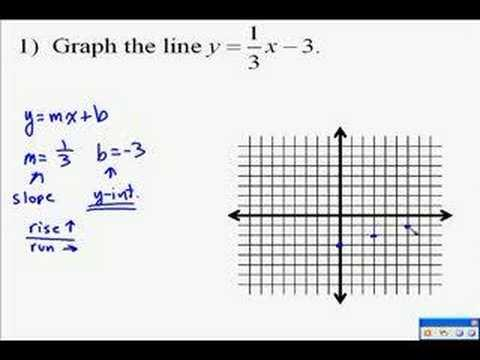# How Well Do You Know Linear Equations & Graphs?

10 Questions | Attempts: 424
ShareSettingsTo graph a linear equation, we can use the slope and y-intercept. Locate the y-intercept on the graph and plot the point. From this point, use the slope to find a second point and plot it. Draw the line that connects the two points.Back to top